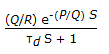Chemical Engineering - Process Control and Instrumentation

Exercise :: Process Control and Instrumentation - Section 3

46.

Which of the following controllers has maximum offset ?

 A. P-controller B. P-I controller C. P-D controller D. P-I-D controller

Explanation:

No answer description available for this question. Let us discuss.

47.

Pressure of 0.01 psi (absolute) can be measured by __________ gauge.

 A. ionisation B. Pirani C. Mcloid D. none of these

Explanation:

No answer description available for this question. Let us discuss.

48.

High temperature of around 2000°C of incandescent gas mantles is measured by a/an

 A. specially designed thermocouple. B. optical pyrometer. C. radiation pyrometer. D. none of these.

Explanation:

No answer description available for this question. Let us discuss.

49.

The unit step response of the transfer function 1/(s2 + 2s + 3)

 A. has a non-zero slope at the origin. B. has a damped oscillatory characteristics. C. is overdamped D. is unstable

Explanation:

No answer description available for this question. Let us discuss.

50.

The experimental response of the controlled variable y(t) for a step change of magnitude P in the manipulated variable x(t) is shown below :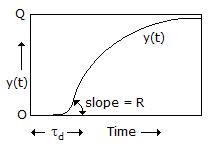The appropriate transfer function of the process is

 A.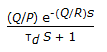B.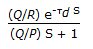C.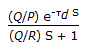D.